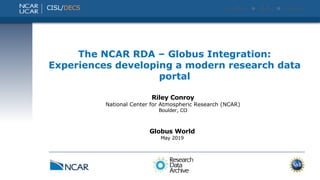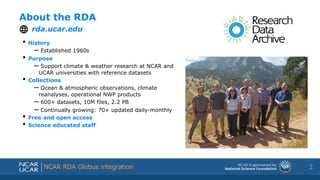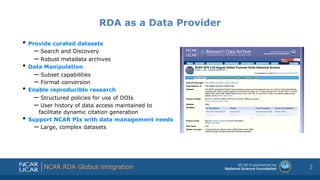Seu SlideShare está sendo baixado. ×

# The NCAR RDA–Globus Integration: Experiences Developing a Modern Research Data Portal

Anúncio
Anúncio
Anúncio
Anúncio
Anúncio
Anúncio
Anúncio
Anúncio
Anúncio
Anúncio
AnúncioCarregando em…3
×

1 de 18 Anúncio

# The NCAR RDA–Globus Integration: Experiences Developing a Modern Research Data Portal

This presentation was given at the 2019 GlobusWorld Conference in Chicago, IL by Riley Conroy from the National Center for Atmospheric Research (NCAR).

This presentation was given at the 2019 GlobusWorld Conference in Chicago, IL by Riley Conroy from the National Center for Atmospheric Research (NCAR).

Anúncio
Anúncio

### The NCAR RDA–Globus Integration: Experiences Developing a Modern Research Data Portal

1. 1. Shortened presentation title Shortened presentation title NCAR RDA Globus integration The NCAR RDA – Globus Integration: Experiences developing a modern research data portal Globus World May 2019 Riley Conroy National Center for Atmospheric Research (NCAR) Boulder, CO CISL/DECS
2. 2. Shortened presentation title Shortened presentation title NCAR RDA Globus integration About the RDA rda.ucar.edu 2 • History – Established 1960s • Purpose – Support climate & weather research at NCAR and UCAR universities with reference datasets • Collections – Ocean & atmospheric observations, climate reanalyses, operational NWP products – 600+ datasets, 10M files, 2.2 PB – Continually growing: 70+ updated daily-monthly • Free and open access • Science educated staff
3. 3. Shortened presentation title Shortened presentation title NCAR RDA Globus integration RDA as a Data Provider • Provide curated datasets – Search and Discovery – Robust metadata archives • Data Manipulation – Subset capabilities – Format conversion • Enable reproducible research – Structured policies for use of DOIs – User history of data access maintained to facilitate dynamic citation generation • Support NCAR PIs with data management needs – Large, complex datasets 3
4. 4. Shortened presentation title Shortened presentation title NCAR RDA Globus integration Good Old Days • Apache directory listing • FTP • cURL/wget 4
5. 5. Shortened presentation title Shortened presentation title NCAR RDA Globus integration Unique Users by Country (Past Year) 5
6. 6. Shortened presentation title Shortened presentation title NCAR RDA Globus integration RDA Data usage • FY 2018 14K+ unique web users 2.85 PB data delivered 6
7. 7. Shortened presentation title Shortened presentation title NCAR RDA Globus integration Challenges 7
8. 8. Shortened presentation title Shortened presentation title NCAR RDA Globus integration Challenges • Authentication –Complicated scripts 8 (?:(?:rn)?[ t])*(?:(?:(?:[^()<>@,;:".[] 000-031]+(?:(?:(?:rn)?[ t] )+|Z|(?=[["()<>@,;:".[]]))|"(?:[^"r]|.|(?:(?:rn)?[ t]))*"(?:(?: rn)?[ t])*)(?:.(?:(?:rn)?[ t])*(?:[^()<>@,;:".[] 000-031]+(?:(?:( ?:rn)?[ t])+|Z|(?=[["()<>@,;:".[]]))|"(?:[^"r]|.|(?:(?:rn)?[ t]))*"(?:(?:rn)?[ t])*))*@(?:(?:rn)?[ t])*(?:[^()<>@,;:".[] 000-0 31]+(?:(?:(?:rn)?[ t])+|Z|(?=[["()<>@,;:".[]]))|[([^[]r]|.)* ](?:(?:rn)?[ t])*)(?:.(?:(?:rn)?[ t])*(?:[^()<>@,;:".[] 000-031]+ (?:(?:(?:rn)?[ t])+|Z|(?=[["()<>@,;:".[]]))|[([^[]r]|.)*](?: (?:rn)?[ t])*))*|(?:[^()<>@,;:".[] 000-031]+(?:(?:(?:rn)?[ t])+|Z |(?=[["()<>@,;:".[]]))|"(?:[^"r]|.|(?:(?:rn)?[ t]))*"(?:(?:rn) ?[ t])*)*<(?:(?:rn)?[ t])*(?:@(?:[^()<>@,;:".[] 000-031]+(?:(?:(?: rn)?[ t])+|Z|(?=[["()<>@,;:".[]]))|[([^[]r]|.)*](?:(?:rn)?[ t])*)(?:.(?:(?:rn)?[ t])*(?:[^()<>@,;:".[] 000-031]+(?:(?:(?:rn) ?[ t])+|Z|(?=[["()<>@,;:".[]]))|[([^[]r]|.)*](?:(?:rn)?[ t] )*))*(?:,@(?:(?:rn)?[ t])*(?:[^()<>@,;:".[] 000-031]+(?:(?:(?:rn)?[ t])+|Z|(?=[["()<>@,;:".[]]))|[([^[]r]|.)*](?:(?:rn)?[ t])* )(?:.(?:(?:rn)?[ t])*(?:[^()<>@,;:".[] 000-031]+(?:(?:(?:rn)?[ t] )+|Z|(?=[["()<>@,;:".[]]))|[([^[]r]|.)*](?:(?:rn)?[ t])*))*) *:(?:(?:rn)?[ t])*)?(?:[^()<>@,;:".[] 000-031]+(?:(?:(?:rn)?[ t])+ |Z|(?=[["()<>@,;:".[]]))|"(?:[^"r]|.|(?:(?:rn)?[ t]))*"(?:(?:r n)?[ t])*)(?:.(?:(?:rn)?[ t])*(?:[^()<>@,;:".[] 000-031]+(?:(?:(?: rn)?[ t])+|Z|(?=[["()<>@,;:".[]]))|"(?:[^"r]|.|(?:(?:rn)?[ t ]))*"(?:(?:rn)?[ t])*))*@(?:(?:rn)?[ t])*(?:[^()<>@,;:".[] 000-031 ]+(?:(?:(?:rn)?[ t])+|Z|(?=[["()<>@,;:".[]]))|[([^[]r]|.)*]( ?:(?:rn)?[ t])*)(?:.(?:(?:rn)?[ t])*(?:[^()<>@,;:".[] 000-031]+(? :(?:(?:rn)?[ t])+|Z|(?=[["()<>@,;:".[]]))|[([^[]r]|.)*](?:(? :rn)?[ t])*))*>(?:(?:rn)?[ t])*)|(?:[^()<>@,;:".[] 000-031]+(?:(? :(?:rn)?[ t])+|Z|(?=[["()<>@,;:".[]]))|"(?:[^"r]|.|(?:(?:rn)? [ t]))*"(?:(?:rn)?[ t])*)*:(?:(?:rn)?[ t])*(?:(?:(?:[^()<>@,;:".[] 000-031]+(?:(?:(?:rn)?[ t])+|Z|(?=[["()<>@,;:".[]]))|"(?:[^"r]| .|(?:(?:rn)?[ t]))*"(?:(?:rn)?[ t])*)(?:.(?:(?:rn)?[ t])*(?:[^()<> @,;:".[] 000-031]+(?:(?:(?:rn)?[ t])+|Z|(?=[["()<>@,;:".[]]))|" (?:[^"r]|.|(?:(?:rn)?[ t]))*"(?:(?:rn)?[ t])*))*@(?:(?:rn)?[ t] )*(?:[^()<>@,;:".[] 000-031]+(?:(?:(?:rn)?[ t])+|Z|(?=[["()<>@,;: ".[]]))|[([^[]r]|.)*](?:(?:rn)?[ t])*)(?:.(?:(?:rn)?[ t])*(? :[^()<>@,;:".[] 000-031]+(?:(?:(?:rn)?[ t])+|Z|(?=[["()<>@,;:".[ ]]))|[([^[]r]|.)*](?:(?:rn)?[ t])*))*|(?:[^()<>@,;:".[] 000- 031]+(?:(?:(?:rn)?[ t])+|Z|(?=[["()<>@,;:".[]]))|"(?:[^"r]|.|( ?:(?:rn)?[ t]))*"(?:(?:rn)?[ t])*)*<(?:(?:rn)?[ t])*(?:@(?:[^()<>@,; :".[] 000-031]+(?:(?:(?:rn)?[ t])+|Z|(?=[["()<>@,;:".[]]))|[([ ^[]r]|.)*](?:(?:rn)?[ t])*)(?:.(?:(?:rn)?[ t])*(?:[^()<>@,;:" .[] 000-031]+(?:(?:(?:rn)?[ t])+|Z|(?=[["()<>@,;:".[]]))|[([^[ ]r]|.)*](?:(?:rn)?[ t])*))*(?:,@(?:(?:rn)?[ t])*(?:[^()<>@,;:". [] 000-031]+(?:(?:(?:rn)?[ t])+|Z|(?=[["()<>@,;:".[]]))|[([^[] r]|.)*](?:(?:rn)?[ t])*)(?:.(?:(?:rn)?[ t])*(?:[^()<>@,;:".[] 000-031]+(?:(?:(?:rn)?[ t])+|Z|(?=[["()<>@,;:".[]]))|[([^[]r] |.)*](?:(?:rn)?[ t])*))*)*:(?:(?:rn)?[ t])*)?(?:[^()<>@,;:".[] 0 00-031]+(?:(?:(?:rn)?[ t])+|Z|(?=[["()<>@,;:".[]]))|"(?:[^"r]| .|(?:(?:rn)?[ t]))*"(?:(?:rn)?[ t])*)(?:.(?:(?:rn)?[ t])*(?:[^()<>@, ;:".[] 000-031]+(?:(?:(?:rn)?[ t])+|Z|(?=[["()<>@,;:".[]]))|"(? :[^"r]|.|(?:(?:rn)?[ t]))*"(?:(?:rn)?[ t])*))*@(?:(?:rn)?[ t])* (?:[^()<>@,;:".[] 000-031]+(?:(?:(?:rn)?[ t])+|Z|(?=[["()<>@,;:". []]))|[([^[]r]|.)*](?:(?:rn)?[ t])*)(?:.(?:(?:rn)?[ t])*(?:[ ^()<>@,;:".[] 000-031]+(?:(?:(?:rn)?[ t])+|Z|(?=[["()<>@,;:".[] ]))|[([^[]r]|.)*](?:(?:rn)?[ t])*))*>(?:(?:rn)?[ t])*)(?:,s*( ?:(?:[^()<>@,;:".[] 000-031]+(?:(?:(?:rn)?[ t])+|Z|(?=[["()<>@,;: ".[]]))|"(?:[^"r]|.|(?:(?:rn)?[ t]))*"(?:(?:rn)?[ t])*)(?:.(?:( ?:rn)?[ t])*(?:[^()<>@,;:".[] 000-031]+(?:(?:(?:rn)?[ t])+|Z|(?=[ ["()<>@,;:".[]]))|"(?:[^"r]|.|(?:(?:rn)?[ t]))*"(?:(?:rn)?[ t ])*))*@(?:(?:rn)?[ t])*(?:[^()<>@,;:".[] 000-031]+(?:(?:(?:rn)?[ t ])+|Z|(?=[["()<>@,;:".[]]))|[([^[]r]|.)*](?:(?:rn)?[ t])*)(? :.(?:(?:rn)?[ t])*(?:[^()<>@,;:".[] 000-031]+(?:(?:(?:rn)?[ t])+| Z|(?=[["()<>@,;:".[]]))|[([^[]r]|.)*](?:(?:rn)?[ t])*))*|(?: [^()<>@,;:".[] 000-031]+(?:(?:(?:rn)?[ t])+|Z|(?=[["()<>@,;:".[ ]]))|"(?:[^"r]|.|(?:(?:rn)?[ t]))*"(?:(?:rn)?[ t])*)*<(?:(?:rn) ?[ t])*(?:@(?:[^()<>@,;:".[] 000-031]+(?:(?:(?:rn)?[ t])+|Z|(?=[[" ()<>@,;:".[]]))|[([^[]r]|.)*](?:(?:rn)?[ t])*)(?:.(?:(?:rn) ?[ t])*(?:[^()<>@,;:".[] 000-031]+(?:(?:(?:rn)?[ t])+|Z|(?=[["()<> @,;:".[]]))|[([^[]r]|.)*](?:(?:rn)?[ t])*))*(?:,@(?:(?:rn)?[ t])*(?:[^()<>@,;:".[] 000-031]+(?:(?:(?:rn)?[ t])+|Z|(?=[["()<>@, ;:".[]]))|[([^[]r]|.)*](?:(?:rn)?[ t])*)(?:.(?:(?:rn)?[ t] )*(?:[^()<>@,;:".[] 000-031]+(?:(?:(?:rn)?[ t])+|Z|(?=[["()<>@,;: ".[]]))|[([^[]r]|.)*](?:(?:rn)?[ t])*))*)*:(?:(?:rn)?[ t])*)? (?:[^()<>@,;:".[] 000-031]+(?:(?:(?:rn)?[ t])+|Z|(?=[["()<>@,;:". []]))|"(?:[^"r]|.|(?:(?:rn)?[ t]))*"(?:(?:rn)?[ t])*)(?:.(?:(?: rn)?[ t])*(?:[^()<>@,;:".[] 000-031]+(?:(?:(?:rn)?[ t])+|Z|(?=[[ "()<>@,;:".[]]))|"(?:[^"r]|.|(?:(?:rn)?[ t]))*"(?:(?:rn)?[ t]) *))*@(?:(?:rn)?[ t])*(?:[^()<>@,;:".[] 000-031]+(?:(?:(?:rn)?[ t]) +|Z|(?=[["()<>@,;:".[]]))|[([^[]r]|.)*](?:(?:rn)?[ t])*)(?: .(?:(?:rn)?[ t])*(?:[^()<>@,;:".[] 000-031]+(?:(?:(?:rn)?[ t])+|Z |(?=[["()<>@,;:".[]]))|[([^[]r]|.)*](?:(?:rn)?[ t])*))*>(?:( ?:rn)?[ t])*))*)?;s*) Email validation regex
9. 9. Shortened presentation title Shortened presentation title NCAR RDA Globus integration Challenges • Authentication –Complicated scripts • Single web server –Limit number of concurrent downloads –Server can become overwhelmed with high use 9 Start of semester (dramatized)
10. 10. Shortened presentation title Shortened presentation title NCAR RDA Globus integration Challenges • Authentication –Complicated scripts • Single web server –Limit number of concurrent downloads –Server can become overwhelmed with high use • User sophistication 10
11. 11. Shortened presentation title Shortened presentation title NCAR RDA Globus integration Challenges • Authentication –Complicated scripts • Single web server –Limit number of concurrent downloads –Server can become overwhelmed with high use • User sophistication • Existing data portal 11
12. 12. Shortened presentation title Shortened presentation title NCAR RDA Globus integration Existing Infrastructure • Existing Globus GridFTP infrastructure in place at NCAR –Science DMZ • 4 servers –100 Gb Ethernet connections –50 GB/s (400 Gb/s) site bandwidth –25 GB/s (200 Gb/s) offsite bandwidth 12
13. 13. Shortened presentation title Shortened presentation title NCAR RDA Globus integration Initial Integration • Determining what was needed • Endpoint management • PHP + Perl scripts • CLI to create and manage shares 13
14. 14. Shortened presentation title Shortened presentation title NCAR RDA Globus integration Further Developments • Shared endpoints –Two permanent shared endpoints •Requests (deleted after 5 days) •Semi-permanent shares • Users added to endpoint ACL –1000 ACLs per endpoint • Database integration –Shares –Manage ACLs 14
15. 15. Shortened presentation title Shortened presentation title NCAR RDA Globus integration 5 Oauth 2.0 Delegated transfers • Curated file lists • Automated transfer of custom delayed mode products
16. 16. Shortened presentation title Shortened presentation title NCAR RDA Globus integration • Delegated OAuth 2.0 transfers –RDA submits transfers on behalf of users –Curated file lists –Automated transfers for delayed mode requests • Authentication with RDA identity –https://github.com/NCAR/rda-globus-myproxy-oauth • Repository –https://github.com/NCAR/rda-globus • Future –HTTP downloads –S3 Connector 16 Recent and Planned Enhancements 4.5 years in operation 4,800 unique users 8,890 data shares 34,400,000 files transferred 1.25 petabytes moved 39 countries (through March 2019) RDA-Globus by the numbers
17. 17. Shortened presentation title Shortened presentation title NCAR RDA Globus integration 17 Testimonial
18. 18. Shortened presentation title Shortened presentation title NCAR RDA Globus integration Questions 18